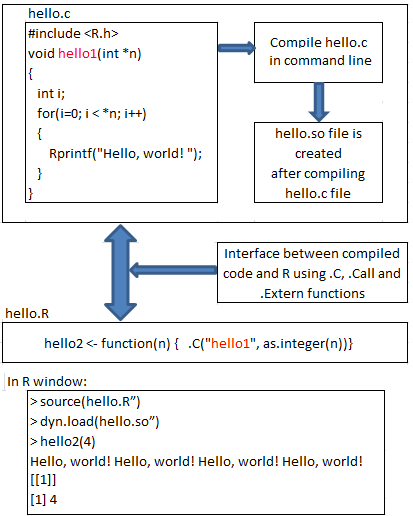Calling C from R programming

PrevNext

• R is a programming language which provides statistical and graphical techniques for data analysis.
• C functions can be called from R programming which is described in detail below.

1. What is R?
2. Purpose of calling C from R programming language
3. Methods of calling C functions from R
5. Example program for Calling C from R using .C

1. What is R?

• R is a programming language which provides statistical, graphical techniques for data analysis.
• R is an interactive, open source and object oriented programming language designed for statisticians.
• R is used to perform data analysis by using scripts and functions which is available in R programming language.
• Complete data analysis could be done  in few lines of code using R programming.

2. Purpose of calling C from R programming language:

• R programming language is slow in iterative algorithm. Iterating very large data sets leads to poor performance in R.
• To improve the performance of R, C functions are called to make use of high performance of C.

3. Methods of calling C functions from R:

• The link/interface between C compiled code and R language is made by below 3 functions.
• These functions are used to call a C function from R. They are,
1. .C
2. .Call
3. .External

• Create a C function with below pre-requisite.
• Prerequisite:

a)      Data type of the function should be void.
b)      Compiled code should not return any value except its arguments.

• Below is the command to compile C code from command prompt. Once function is written in C, use below command to compile.

R CMD SHLIB -lgsl –lgslcblas test.c

• After compiling, we get the compiled code file name as test.so
• Then, this compiled code should be loaded in running R session using the  command dyn.load(“test.so”)
• Once above dynamic load is done, all the functions written in C file will be available for R to call.

5. Example program using .C

• Please consider below file “hello.c” in which a C function “hello” is written.
• Please note that we should not use main function as we are not going to write C program. We are calling a C function from R.

File name:       hello.c

• Below file “hello.R” is the R file from where C function “hello1” is called.

File name:       hello.R

• Now, compile “hello.c” file using below command.

R CMD SHLIB -lgsl –lgslcblas test.c

• After compiling hello.c file using above command, hello.so file is created which is loaded in running R session using the below command dyn.load(“hello.so”)
• Now, we launch R application and type the below as mentioned in above steps.

In R:

 > source(hello.R”) > dyn.load(hello.so”) > hello2(4) Hello, world! Hello, world! Hello, world! Hello, world! []  4

Pictorial representation for calling C from R programming: